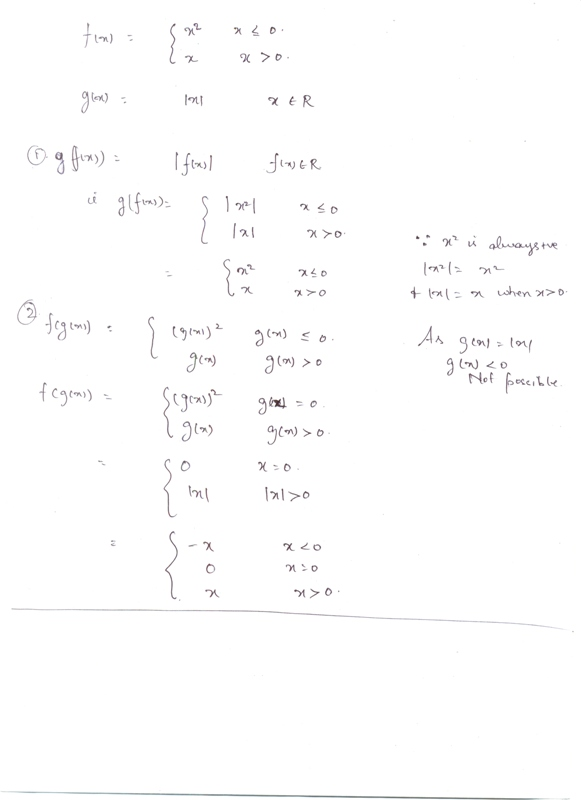# If f(x)=x2 when x≤0         =x  when x>0,and g(x)=-ιxι,x belongs to R,then find fog and gof.Here ιxι denotes modulus of x.11 years ago

fog(x)=f(x) for x≥0 and is undefined for x<0

gof(x)=f(x), which is obvious

11 years ago

Hi Menka,

The solution is in the scanned copy.Hope that helps.

All the best.

Regards,